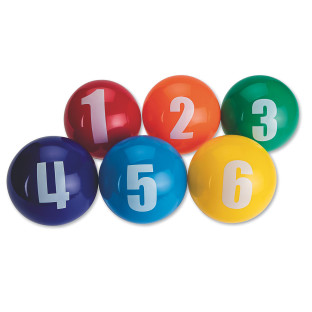Credit for this problem goes to jlevinson. Thanks!

## All wrongI have 8 balls, numbered 1-8, and 8 boxes, numbered 1-8. I randomly put one ball in each box.

What’s the probability that I never put a ball numbered $$x$$ into a box with the same number $$x$$?

Solution